# Poisson equation

(diff) ← Older revision | Latest revision (diff) | Newer revision → (diff)
A partial differential equation which is satisfied by the potential of a mass distribution inside domains occupied by the masses creating this potential. For the Newton potential in the space,, and the logarithmic potential in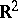the Poisson equation has the form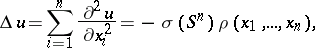where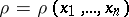is the density of the mass distribution,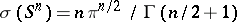is the area of the unit sphere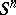inand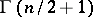is the value of the gamma-function.